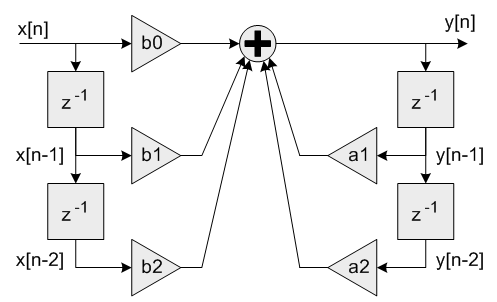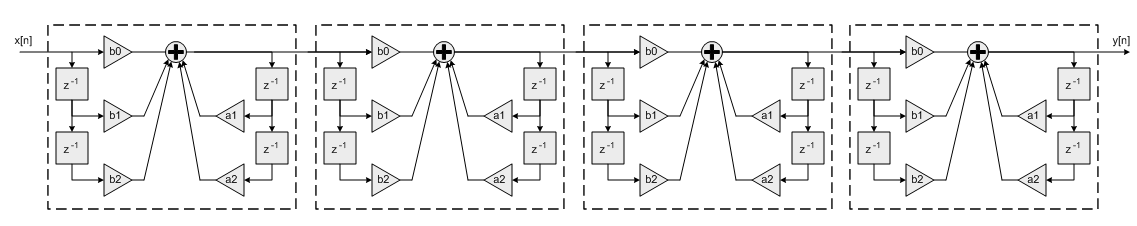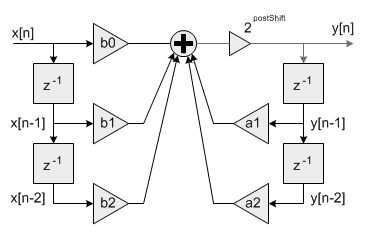CMSIS-DSP  Version 1.9.0 CMSIS DSP Software Library

Description

This set of functions implements arbitrary order recursive (IIR) filters. The filters are implemented as a cascade of second order Biquad sections. The functions support Q15, Q31 and floating-point data types. Fast version of Q15 and Q31 also available.

The functions operate on blocks of input and output data and each call to the function processes blockSize samples through the filter. pSrc points to the array of input data and pDst points to the array of output data. Both arrays contain blockSize values.

Algorithm
Each Biquad stage implements a second order filter using the difference equation:
y[n] = b0 * x[n] + b1 * x[n-1] + b2 * x[n-2] + a1 * y[n-1] + a2 * y[n-2]
A Direct Form I algorithm is used with 5 coefficients and 4 state variables per stage.Coefficients b0, b1 and b2 multiply the input signal x[n] and are referred to as the feedforward coefficients. Coefficients a1 and a2 multiply the output signal y[n] and are referred to as the feedback coefficients. Pay careful attention to the sign of the feedback coefficients. Some design tools use the difference equation
y[n] = b0 * x[n] + b1 * x[n-1] + b2 * x[n-2] - a1 * y[n-1] - a2 * y[n-2]
In this case the feedback coefficients a1 and a2 must be negated when used with the CMSIS DSP Library.
Higher order filters are realized as a cascade of second order sections. numStages refers to the number of second order stages used. For example, an 8th order filter would be realized with numStages=4 second order stages.A 9th order filter would be realized with numStages=5 second order stages with the coefficients for one of the stages configured as a first order filter (b2=0 and a2=0).
The pState points to state variables array. Each Biquad stage has 4 state variables x[n-1], x[n-2], y[n-1], and y[n-2]. The state variables are arranged in the pState array as:
{x[n-1], x[n-2], y[n-1], y[n-2]}
The 4 state variables for stage 1 are first, then the 4 state variables for stage 2, and so on. The state array has a total length of 4*numStages values. The state variables are updated after each block of data is processed, the coefficients are untouched.
Instance Structure
The coefficients and state variables for a filter are stored together in an instance data structure. A separate instance structure must be defined for each filter. Coefficient arrays may be shared among several instances while state variable arrays cannot be shared. There are separate instance structure declarations for each of the 3 supported data types.
Init Function
There is also an associated initialization function for each data type. The initialization function performs following operations:
• Sets the values of the internal structure fields.
• Zeros out the values in the state buffer. To do this manually without calling the init function, assign the follow subfields of the instance structure: numStages, pCoeffs, pState. Also set all of the values in pState to zero.
Use of the initialization function is optional. However, if the initialization function is used, then the instance structure cannot be placed into a const data section. To place an instance structure into a const data section, the instance structure must be manually initialized. Set the values in the state buffer to zeros before static initialization. The code below statically initializes each of the 3 different data type filter instance structures
arm_biquad_casd_df1_inst_f32 S1 = {numStages, pState, pCoeffs};
arm_biquad_casd_df1_inst_q15 S2 = {numStages, pState, pCoeffs, postShift};
arm_biquad_casd_df1_inst_q31 S3 = {numStages, pState, pCoeffs, postShift};
where numStages is the number of Biquad stages in the filter; pState is the address of the state buffer; pCoeffs is the address of the coefficient buffer; postShift shift to be applied.
Fixed-Point Behavior
Care must be taken when using the Q15 and Q31 versions of the Biquad Cascade filter functions. Following issues must be considered:
• Scaling of coefficients
• Filter gain
• Overflow and saturation
Scaling of coefficients
Filter coefficients are represented as fractional values and coefficients are restricted to lie in the range [-1 +1). The fixed-point functions have an additional scaling parameter postShift which allow the filter coefficients to exceed the range [+1 -1). At the output of the filter's accumulator is a shift register which shifts the result by postShift bits.Fixed-point Biquad with shift by postShift bits after accumulator
This essentially scales the filter coefficients by 2^postShift. For example, to realize the coefficients
{1.5, -0.8, 1.2, 1.6, -0.9}
set the pCoeffs array to:
{0.75, -0.4, 0.6, 0.8, -0.45}
and set postShift=1
Filter gain
The frequency response of a Biquad filter is a function of its coefficients. It is possible for the gain through the filter to exceed 1.0 meaning that the filter increases the amplitude of certain frequencies. This means that an input signal with amplitude < 1.0 may result in an output > 1.0 and these are saturated or overflowed based on the implementation of the filter. To avoid this behavior the filter needs to be scaled down such that its peak gain < 1.0 or the input signal must be scaled down so that the combination of input and filter are never overflowed.
Overflow and saturation
For Q15 and Q31 versions, it is described separately as part of the function specific documentation below.

Function Documentation

 void arm_biquad_cascade_df1_f16 ( const arm_biquad_casd_df1_inst_f16 * S, const float16_t * pSrc, float16_t * pDst, uint32_t blockSize )
Parameters
 [in] S points to an instance of the floating-point Biquad cascade structure [in] pSrc points to the block of input data [out] pDst points to the block of output data [in] blockSize number of samples to process
Returns
none
 void arm_biquad_cascade_df1_f32 ( const arm_biquad_casd_df1_inst_f32 * S, const float32_t * pSrc, float32_t * pDst, uint32_t blockSize )
Parameters
 [in] S points to an instance of the floating-point Biquad cascade structure [in] pSrc points to the block of input data [out] pDst points to the block of output data [in] blockSize number of samples to process
Returns
none
 void arm_biquad_cascade_df1_fast_q15 ( const arm_biquad_casd_df1_inst_q15 * S, const q15_t * pSrc, q15_t * pDst, uint32_t blockSize )

Fast but less precise processing function for the Q15 Biquad cascade filter for Cortex-M3 and Cortex-M4.

Parameters
 [in] S points to an instance of the Q15 Biquad cascade structure [in] pSrc points to the block of input data [out] pDst points to the block of output data [in] blockSize number of samples to process per call
Returns
none
Scaling and Overflow Behavior
This fast version uses a 32-bit accumulator with 2.30 format. The accumulator maintains full precision of the intermediate multiplication results but provides only a single guard bit. Thus, if the accumulator result overflows it wraps around and distorts the result. In order to avoid overflows completely the input signal must be scaled down by two bits and lie in the range [-0.25 +0.25). The 2.30 accumulator is then shifted by postShift bits and the result truncated to 1.15 format by discarding the low 16 bits.
Remarks
Refer to arm_biquad_cascade_df1_q15() for a slower implementation of this function which uses 64-bit accumulation to avoid wrap around distortion. Both the slow and the fast versions use the same instance structure. Use the function arm_biquad_cascade_df1_init_q15() to initialize the filter structure.
 void arm_biquad_cascade_df1_fast_q31 ( const arm_biquad_casd_df1_inst_q31 * S, const q31_t * pSrc, q31_t * pDst, uint32_t blockSize )

Fast but less precise processing function for the Q31 Biquad cascade filter for Cortex-M3 and Cortex-M4.

Parameters
 [in] S points to an instance of the Q31 Biquad cascade structure [in] pSrc points to the block of input data [out] pDst points to the block of output data [in] blockSize number of samples to process per call
Returns
none
Scaling and Overflow Behavior
This function is optimized for speed at the expense of fixed-point precision and overflow protection. The result of each 1.31 x 1.31 multiplication is truncated to 2.30 format. These intermediate results are added to a 2.30 accumulator. Finally, the accumulator is saturated and converted to a 1.31 result. The fast version has the same overflow behavior as the standard version and provides less precision since it discards the low 32 bits of each multiplication result. In order to avoid overflows completely the input signal must be scaled down by two bits and lie in the range [-0.25 +0.25). Use the intialization function arm_biquad_cascade_df1_init_q31() to initialize filter structure.
Remarks
Refer to arm_biquad_cascade_df1_q31() for a slower implementation of this function which uses 64-bit accumulation to provide higher precision. Both the slow and the fast versions use the same instance structure. Use the function arm_biquad_cascade_df1_init_q31() to initialize the filter structure.
Parameters
 [in,out] S points to an instance of the floating-point Biquad cascade structure. [in] numStages number of 2nd order stages in the filter. [in] pCoeffs points to the filter coefficients. [in] pState points to the state buffer.
Returns
none
Coefficient and State Ordering
The coefficients are stored in the array pCoeffs in the following order:
{b10, b11, b12, a11, a12, b20, b21, b22, a21, a22, ...}
where b1x and a1x are the coefficients for the first stage, b2x and a2x are the coefficients for the second stage, and so on. The pCoeffs array contains a total of 5*numStages values.
The pState is a pointer to state array. Each Biquad stage has 4 state variables x[n-1], x[n-2], y[n-1], and y[n-2]. The state variables are arranged in the pState array as:
{x[n-1], x[n-2], y[n-1], y[n-2]}
The 4 state variables for stage 1 are first, then the 4 state variables for stage 2, and so on. The state array has a total length of 4*numStages values. The state variables are updated after each block of data is processed; the coefficients are untouched.
For MVE code, an additional buffer of modified coefficients is required.
Its size is numStages and each element of this buffer has type arm_biquad_mod_coef_f16. So, its total size is 96*numStages float16_t elements.

Parameters
 [in,out] S points to an instance of the floating-point Biquad cascade structure. [in] numStages number of 2nd order stages in the filter. [in] pCoeffs points to the filter coefficients. [in] pState points to the state buffer.
Returns
none
Coefficient and State Ordering
The coefficients are stored in the array pCoeffs in the following order:
{b10, b11, b12, a11, a12, b20, b21, b22, a21, a22, ...}
where b1x and a1x are the coefficients for the first stage, b2x and a2x are the coefficients for the second stage, and so on. The pCoeffs array contains a total of 5*numStages values.
The pState is a pointer to state array. Each Biquad stage has 4 state variables x[n-1], x[n-2], y[n-1], and y[n-2]. The state variables are arranged in the pState array as:
{x[n-1], x[n-2], y[n-1], y[n-2]}
The 4 state variables for stage 1 are first, then the 4 state variables for stage 2, and so on. The state array has a total length of 4*numStages values. The state variables are updated after each block of data is processed; the coefficients are untouched.
For MVE code, an additional buffer of modified coefficients is required.
Its size is numStages and each element of this buffer has type arm_biquad_mod_coef_f32. So, its total size is 32*numStages float32_t elements.

 void arm_biquad_cascade_df1_init_q15 ( arm_biquad_casd_df1_inst_q15 * S, uint8_t numStages, const q15_t * pCoeffs, q15_t * pState, int8_t postShift )
Parameters
 [in,out] S points to an instance of the Q15 Biquad cascade structure. [in] numStages number of 2nd order stages in the filter. [in] pCoeffs points to the filter coefficients. [in] pState points to the state buffer. [in] postShift Shift to be applied to the accumulator result. Varies according to the coefficients format
Returns
none
Coefficient and State Ordering
The coefficients are stored in the array pCoeffs in the following order:
{b10, 0, b11, b12, a11, a12, b20, 0, b21, b22, a21, a22, ...}
where b1x and a1x are the coefficients for the first stage, b2x and a2x are the coefficients for the second stage, and so on. The pCoeffs array contains a total of 6*numStages values. The zero coefficient between b1 and b2 facilities use of 16-bit SIMD instructions on the Cortex-M4.
The state variables are stored in the array pState. Each Biquad stage has 4 state variables x[n-1], x[n-2], y[n-1], and y[n-2]. The state variables are arranged in the pState array as:
{x[n-1], x[n-2], y[n-1], y[n-2]}
The 4 state variables for stage 1 are first, then the 4 state variables for stage 2, and so on. The state array has a total length of 4*numStages values. The state variables are updated after each block of data is processed; the coefficients are untouched.
 void arm_biquad_cascade_df1_init_q31 ( arm_biquad_casd_df1_inst_q31 * S, uint8_t numStages, const q31_t * pCoeffs, q31_t * pState, int8_t postShift )
Parameters
 [in,out] S points to an instance of the Q31 Biquad cascade structure. [in] numStages number of 2nd order stages in the filter. [in] pCoeffs points to the filter coefficients. [in] pState points to the state buffer. [in] postShift Shift to be applied after the accumulator. Varies according to the coefficients format
Returns
none
Coefficient and State Ordering
The coefficients are stored in the array pCoeffs in the following order:
{b10, b11, b12, a11, a12, b20, b21, b22, a21, a22, ...}
where b1x and a1x are the coefficients for the first stage, b2x and a2x are the coefficients for the second stage, and so on. The pCoeffs array contains a total of 5*numStages values.
The pState points to state variables array. Each Biquad stage has 4 state variables x[n-1], x[n-2], y[n-1], and y[n-2]. The state variables are arranged in the pState array as:
{x[n-1], x[n-2], y[n-1], y[n-2]}
The 4 state variables for stage 1 are first, then the 4 state variables for stage 2, and so on. The state array has a total length of 4*numStages values. The state variables are updated after each block of data is processed; the coefficients are untouched.
 void arm_biquad_cascade_df1_q15 ( const arm_biquad_casd_df1_inst_q15 * S, const q15_t * pSrc, q15_t * pDst, uint32_t blockSize )
Parameters
 [in] S points to an instance of the Q15 Biquad cascade structure [in] pSrc points to the block of input data [out] pDst points to the location where the output result is written [in] blockSize number of samples to process
Returns
none
Scaling and Overflow Behavior
The function is implemented using a 64-bit internal accumulator. Both coefficients and state variables are represented in 1.15 format and multiplications yield a 2.30 result. The 2.30 intermediate results are accumulated in a 64-bit accumulator in 34.30 format. There is no risk of internal overflow with this approach and the full precision of intermediate multiplications is preserved. The accumulator is then shifted by postShift bits to truncate the result to 1.15 format by discarding the low 16 bits. Finally, the result is saturated to 1.15 format.
Remarks
Refer to arm_biquad_cascade_df1_fast_q15() for a faster but less precise implementation of this filter.
 void arm_biquad_cascade_df1_q31 ( const arm_biquad_casd_df1_inst_q31 * S, const q31_t * pSrc, q31_t * pDst, uint32_t blockSize )
Parameters
 [in] S points to an instance of the Q31 Biquad cascade structure [in] pSrc points to the block of input data [out] pDst points to the block of output data [in] blockSize number of samples to process
Returns
none
Scaling and Overflow Behavior
The function is implemented using an internal 64-bit accumulator. The accumulator has a 2.62 format and maintains full precision of the intermediate multiplication results but provides only a single guard bit. Thus, if the accumulator result overflows it wraps around rather than clip. In order to avoid overflows completely the input signal must be scaled down by 2 bits and lie in the range [-0.25 +0.25). After all 5 multiply-accumulates are performed, the 2.62 accumulator is shifted by postShift bits and the result truncated to 1.31 format by discarding the low 32 bits.
Remarks
Refer to arm_biquad_cascade_df1_fast_q31() for a faster but less precise implementation of this filter.